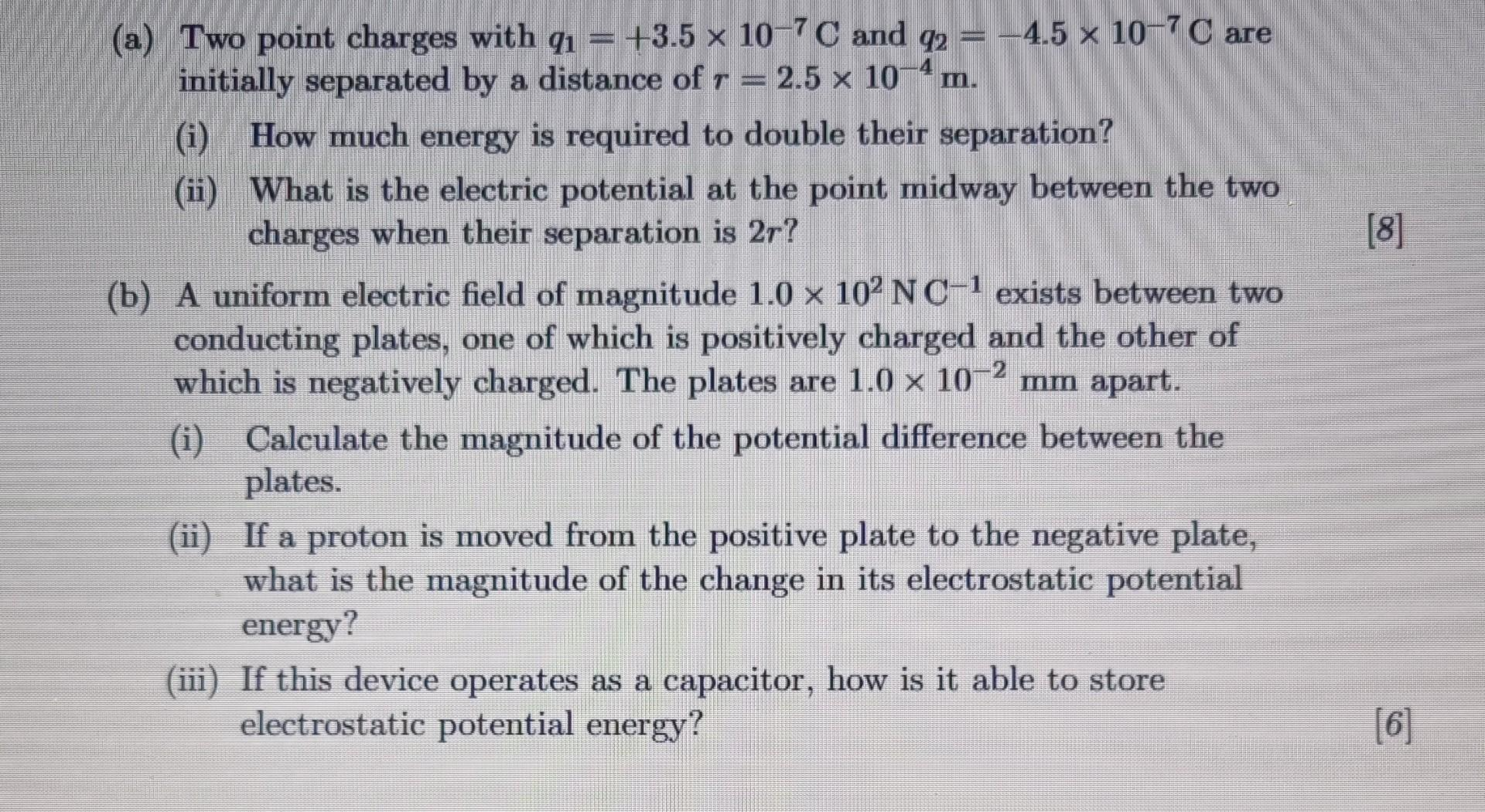Home / Expert Answers / Physics / electric-potential-and-potential-energy-nbsp-a-two-point-charges-with-q-1-3-5-times-1-pa923

# (Solved): electric potential and potential energy   (a) Two point charges with $$q_{1}=+3.5 \times 1 ... electric potential and potential energy(a) Two point charges with \( q_{1}=+3.5 \times 10^{-7} \mathrm{C}$$ and $$q_{2}=-4.5 \times 10^{-7} \mathrm{C}$$ are initially separated by a distance of $$r=2.5 \times 10^{-4} \mathrm{~m}$$. (i) How much energy is required to double their separation? (ii) What is the electric potential at the point midway between the two charges when their separation is $$2 r$$ ? (b) A uniform electric field of magnitude $$1.0 \times 10^{2} \mathrm{~N} \mathrm{C}^{-1}$$ exists between two conducting plates, one of which is positively charged and the other of which is negatively charged. The plates are $$1.0 \times 10^{-2} \mathrm{~mm}$$ apart. (i) Calculate the magnitude of the potential difference between the plates. (ii) If a proton is moved from the positive plate to the negative plate, what is the magnitude of the change in its electrostatic potential energy? (iii) If this device operates as a capacitor, how is it able to store electrostatic potential energy?

We have an Answer from Expert